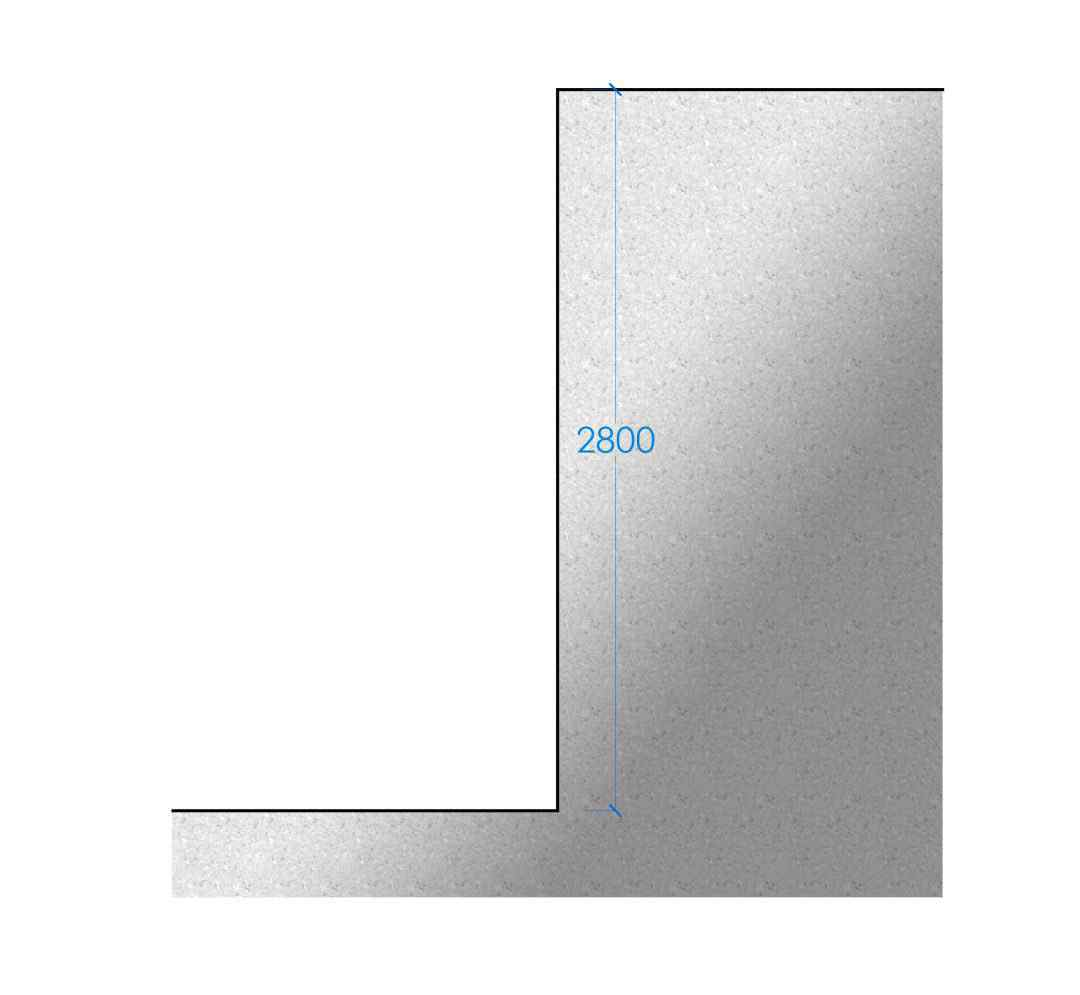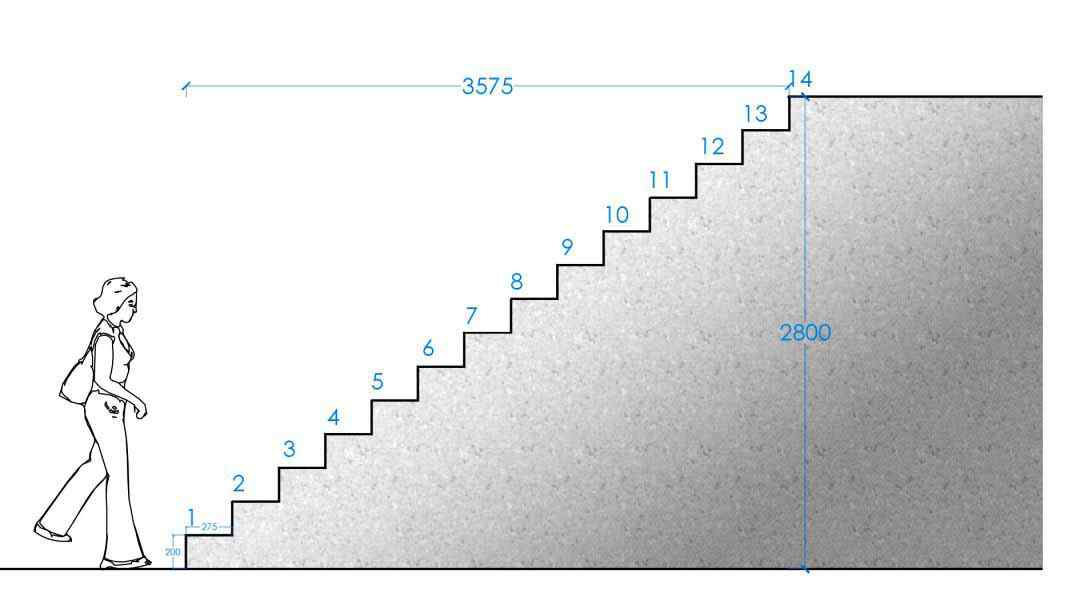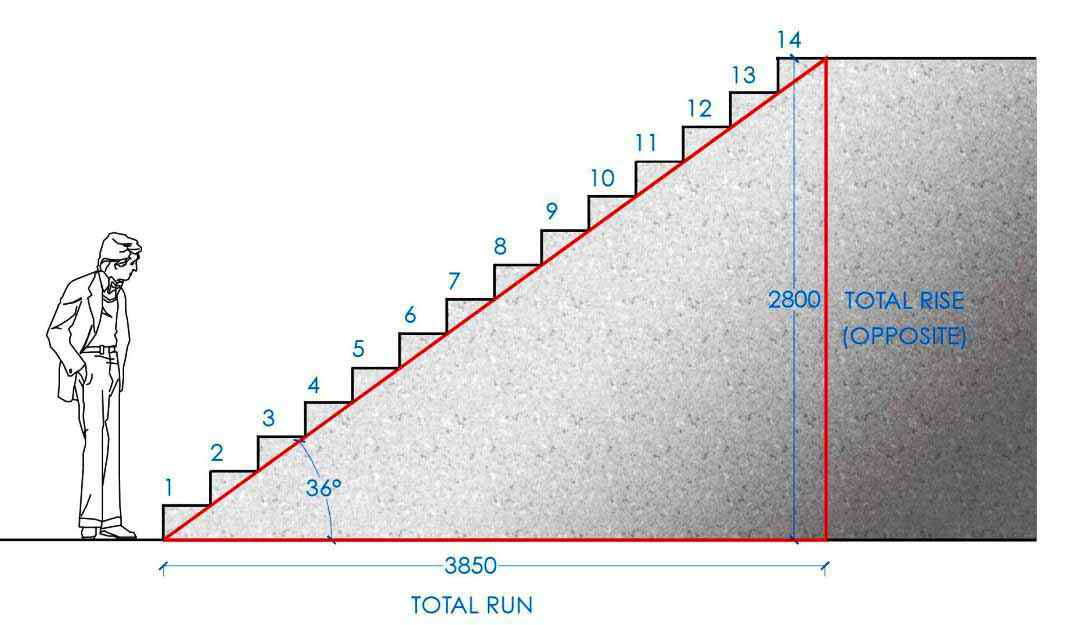# Thumb Rules for Staircase Design Calculation | Concrete Calculation of Staircase | What is Staircase

In this Article today we will talk about the Thumb Rules for Staircase | Stair Formula 2R T | Staircase Cost Calculator | Staircase Design Calculation Example | Staircase Standard Step Height | How to Calculate Concrete for Stairs | Concrete Stairs Design | Standard Staircase Sizes | Standard Stair Tread Thickness

## What is Staircase?

Stairs are used to move from floor to floor. Stairs allow access and communication between floors in multi-story buildings. The room or space between which a stair is located is known as the staircase.

It should therefore be covered with fire-resistant walls, floors, roofs and doors. It should be designed to carry certain loads used for the design of floors. Various materials such as wood, brick, stone, steel or reinforced cement concrete can be used for stair construction. Thumb Rules for Staircase

## Thumb Rules for Staircase Design Calculation:

### Important points to consider when planning the staircase design calculation | Thumb Rules for Stircase

1. Width of Stair: It should not be less than 1 m.
2. Length of flight: The number of steps in a single flight should not be more than 12.
3. Pitch of the stair: It should be between 25 to 40
4. Width of landing: It should be 150mm, more than the width of stair.
5. Length of Step: The length of the step should be at least 600 mm.
6. Winders or kite steps: Odd shaped steps should be avoided and incase found necessary. These should be provided at the start of a stair.
7. Han-rails: It should be 750 to 850 mm in height from the top of respective step or landing.
8. Step proportions: The size of rise and tread in a stair should be kept uniform through out the whole stair.
9. Headroom: Minimum of 2000mm of clear headroom is required above the pitch line
10. Following proportions are recommended:
• Generally: Riser (150 mm to 200 mm) , Tread (250 mm to 300 mm)
• Residential buildings: Tread=250 mm, Riser = 160mm
• Public buildings: Tread=300 mm, Riser= 150mm
• Industrial buildings, Railway station, etc: Tread = not less then 250 mm (250 to 300 mm) , Riser = not less then 150 mm (150 to 190 mm)
11. Otherwise work out the sizes of rise and tread by using any one of the following proportions: Stair Formula 2R T
• (2 x Rise) + (Going), In mm = 550 to 600 mm
• Rise x tread, both in cm = 400 to 410 cm2.
• With basic proportion of going 300 mm and rise 150 mm, add 10 mm to rise for every 20 mm deduction from going e.g. For a going of 280 mm the rise will be 160 mm.

The above rules act as guide but the actual sizes depend upon the availability of space, while planning stairs practical-field. Staircase Cost Calculator

## How to Calculate Number of Riser and Tread for a Staircase Steps | Stair Calculation Formulas:

In this example we will take a Floor to Floor level of 2800mm / 2.80m (Total Rise). Staircase Cost Calculator### 1. Consider the Size of Riser:

Size of riser = 8” (inch) (Range of riser = 6”to 8”)

= 200 mm (Range of riser = 150 mm to 200 mm Generally) Staircase Cost Calculator

### 2. Consider the Size of Tread:

Size of Tread = 11” (inch) (Range of riser = 10”to 1′)

= 275 mm (Range of riser = 250 mm to 300 mm Generally)

Formula, for the size of Tread if Run (Width) is given: So, Size of Tread = Total Run (width) of Stair / Nos. of Thread Staircase Design Calculation Example

### 3. Calculate the Number of Risers:

Number of Riser = Clear Distance between two floors / Size of Riser

= (2800 / 200)

= 14 nos.

Now, we will calculate the size of tread, Staircase Design Calculation Example

### 4. Calculate the Number of Treads:

We know that for any stair numbers of tread for any single flight are one less than several rises.)

Nos of threads on single flight = Nos. of Rising of single flight – 1

= 14 – 1

= 13 nos. Staircase Standard Step Height

### 5. Calculate the Width of Run:

So, The Total Run of Stair = Size of Tread x (Nos. of Threads + 1)

= 275 x 14

Total Run = 3850 mm Staircase Standard Step Height### 6. Calculate the Angle of Stair:

As we know that,

Tan Ɵ = Rise / Run Angle of Stair =

Ɵ = tan-1 (Total Rise / Total Run)

= tan-1 (2800 / 3850)

= tan-1 (0.72)

= 36 Degree

So the required Angle of Stair is 36 Degree  How to Calculate Concrete for Stairs

### 7. Calculate Step Length | Stair Formula 2R+t:

Nicolas Francois Blondel (1675–1683) was the first known person to establish the ergonomic relationship of tread and riser dimensions in the last volume of his Cours d’architecture. How to Calculate Concrete for Stairs

He specified that 2 x riser + tread = step length.

Step Length = 2R + T

but here we consider directly step length = 1.2m How to Calculate Concrete for Stairs

### Final Data: Thumb Rules for Staircase

1. Size of Rizer = 200 mm
2. Size of Tread = 275 mm
3. Total Number of Risers = 14 Nos
4. Total Number of Treads = 13 Nos
5. Width of Total Run = 3850 mm
6. Angle of Stair = 36 Degree

## Concrete Calculation of Staircase

### Section 1: Lannding

• We dont show landing in this example so we consider the size of landing i.e L=1.2m , B=2m and H=0.150m
• Landing area concrete = L x B x H
• Landing area concrete = 1.2 m.  x 2.0 M. x 0.150 M.
• Landing area concrete = 0.36 Cu.m.——–(1)

### Section 2: Waist Slab

• Waist Slab Laenght = (a2+b2)= c2
• C = Lenght of Waist slab =
• A = Hight of Landing Slab = 2.800
• B = Breadth of Wasit slab = 3.850
• Waist Slab Laenght = (2.8002+3.8502)= c2
• Waist Slab Laenght = =√(22.6625)
• Waist Slab Laenght = =4.760 m
• Wasit slab area concrete = L x B x H x N
• Wasit slab area concrete = 4.760 m. x 1.2 m. x 0.150 m. x 1 Nos.
• Wasit slab area concrete = 0.857 Cu.m. ——–(2)

### Section 3: Steps

• Steps area concrete = L x Volume of triangular area x No of Landings x Q
• Steps area concrete = 1.2 m. x ( 0.200 m. x 0.275 m x (1/2)) X 1 X 13 Qty
• Steps area concrete = 0.429 Cu.m. ——–(3)
• Total Concrete of Staircase = (1) + (2) + (3)
• Total Concrete of Staircase = 0.36 Cu.m. + 0.857 Cu.m. + 0.429 Cu.m.
• Total Concrete of Staircase = 1.646 Cu.m.

## FAQs:

### Q. What is the maximum / minimum width of a stair?

For buildings other than dwellings:

• A minimum stair width of 1200mm is acceptable where the stair is between enclosing walls, strings or up stands.
• A minimum width between handrails of 1000mm.
• If the width of the stair is more than 2m wide, the flight must be divided with a handrail to a minimum of 1m wide.

For dwellings:

• 900mm is an acceptable width for stairs. Standard Stair Tread Thickness

### Q. How many steps require a landing?

For general access stairs a maximum allowance of 12 steps before a landing is required. With utility stairs, a maximum of 16 steps is allowed before a landing. In no circumstances should there be a single step, so it is better to balance out the landing position so that there are at least two or more steps either side of the landing.

### Q. What is the minimum height between the stair and ceiling?

It is stipulated in the building regulations that headroom on landings and on the flight of stairs must be at least 2m.

Minimum headroom for a loft conversion can be reduced to 1.8m at its lowest point, but 1.9m at the centre point of the stair. Standard Stair Tread Thickness

### Q. How long can a flight of stairs be?

A flight of stairs with more than 36 risers in consecutive flights a minimum of one change of direction must be included between flights – this applies to both dwellings and buildings other than dwellings. Standard Staircase Sizes

For flights between landings the maximum number of riser should be:

• Utility stairs – 16 risers
• General access stairs – 12 risers Standard Staircase Sizes

### Q. Does a handrail have to be provided?

Yes, in all buildings a handrail must be provided and positioned between 900mm and 1100mm from the pitch line or floor. Standard Staircase Sizes

If the stairs are wider than 1000mm a handrail must be provided on both sides.

### General Notes for Civil Engineering | Standard Data for Civil Engineers

How to Calculate the Volume of Concrete for Staircase

## Conclusion:

1.PROMISEN MBADEE NWATOR
2.Achu cyril Afegenui